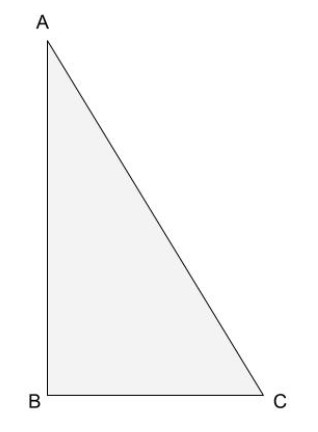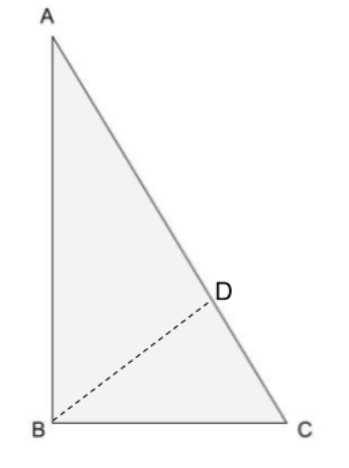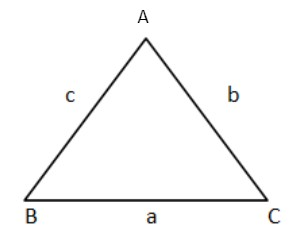QuestionAnswers

# Prove that in any triangle, the side opposite to the larger angle is larger.Verified
128.7k+ views
Hint:We know that the sides opposite to the equal sides are equal. Similarly the side opposite to the larger angle is larger.Using this concept we try prove the statement.

Generally this is the theorem that the side opposite to the larger angle is larger. So now let us see how.
Let $ABC$ be a triangle.Let us assume that $\angle ABC > \angle ACB$
So if we prove that $AC > AB$, then it is clear that the side opposite to the larger angle is larger.
For this proof we need the construction.
So construct $BD$ on $AC$ such that $\angle DBC = \angle ACB$
As $\angle ABC > \angle ACB$, so we can find a point on $AC$ that is $D$ such that $\angle DBC = \angle ACB$.
As construction is done, it divides $\Delta ABC$ into two triangles that are $\Delta ABD$ and $\Delta DBC$.Now in $\Delta DBC$,
$\angle DBC = \angle ACB$
As we constructed $BD$ such that the two angles are equal.
Now we know that in the triangle, the sides opposite to equal angles are equal.
So $BD = DC$ $- - - - - - - \left( 1 \right)$
Now we can add $AD$ on both sides of this above equation,
$AD + BD = DC + AD$
Now if solve $AD + DC$, we get $AC$
So $AD + BD = AC$
Or we can say that,
$AC = BD + AD$ $- - - - - - - \left( 2 \right)$
Now in $\Delta ADB$,
We know that for any triangle to form, the sum of two sides will always be greater than the third side.
So $BD + AD > AB$ $- - - - - - - - \left( 3 \right)$
Now putting the value of $AD + BD = AC$ in equation (3),
So we get $AC > AB$.
Hence it is proved that $AC > AB$ if $\angle ABC > \angle ACB$
Hence we can say that the side opposite to the larger angle is larger.

Note:Let for any triangle $ABC$, by applying the $\sin$ rule we get,$\dfrac{{\sin A}}{a} = \dfrac{{\sin B}}{b} = \dfrac{{\sin C}}{c}$
So if $\angle A > \angle B$,
$\sin A > \sin B$ as $\sin$ is the increasing function.
So $\dfrac{{\sin A}}{a} = \dfrac{{\sin B}}{b}$
$a = \dfrac{{\sin A}}{{\sin B}}b$ and $\sin A > \sin B$
So $a > b$
Hence Proved.